Function Repository Resource:

# PrincipalCurvatures

Compute the principal curvatures of a surface

Contributed by: Wolfram Staff (original content by Alfred Gray)
 ResourceFunction["PrincipalCurvatures"][s,{u,v}] computes the principal curvatures of the surface s with respect to variables u and v. ResourceFunction["PrincipalCurvatures"][eq,{x,y,z}] computes the principal curvatures of the surface given by the implicit equation eq in variables x,y and z.

## Details

The principal curvatures κ1 and κ2 give the maximum and minimum of the normal curvature at a given point on a regular surface and measure the maximum and minimum bending of the surface for every point.
The product of the principal curvatures is known as the Gaussian curvature and the mean of the principal curvatures is known as the mean curvature.

## Examples

### Basic Examples (3)

Define a torus:

 In:=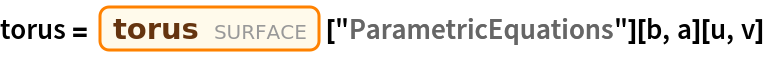Out=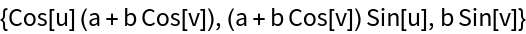Calculate the principal curvatures:

 In:=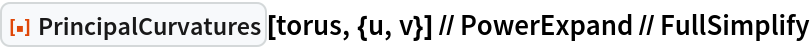Out=The principal curvatures for an implicit surface:

 In:=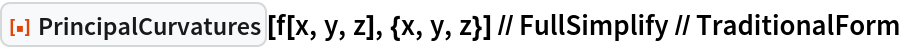Out=Define the implicit Cartesian equation for the ellipsoid:The principal curvatures:

 In:=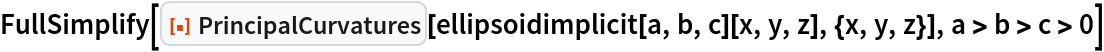Out=In:=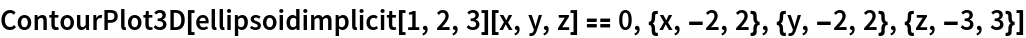Out=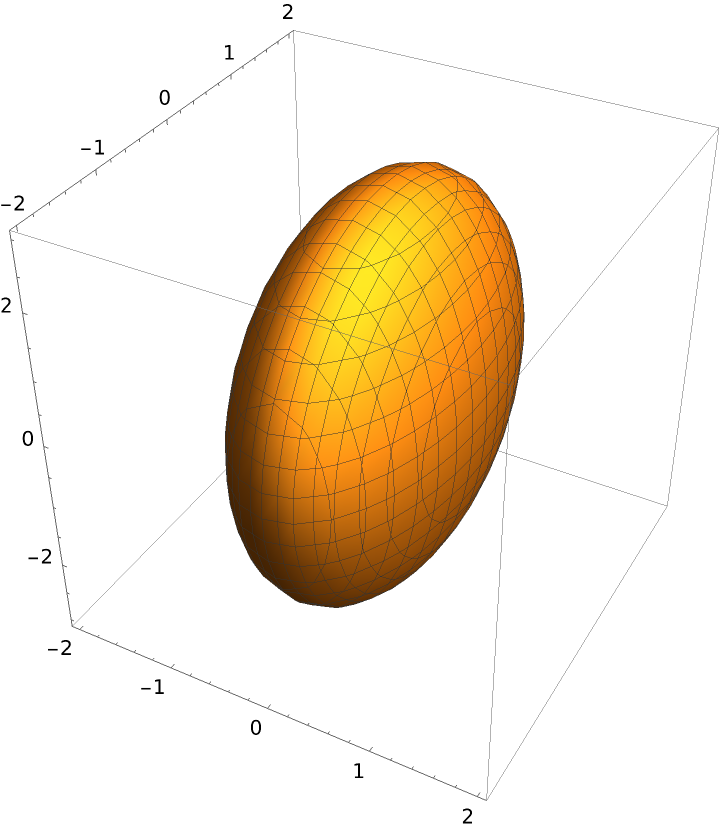### Properties and Relations (5)

Define a surface:

 In:=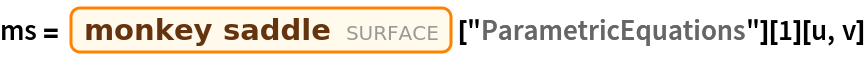Out=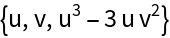Compute the Gaussian curvature and mean curvature:

 In:=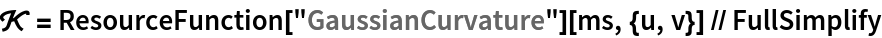Out=In:=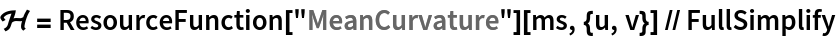Out=In:=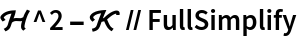Out=Principal curvatures are equal to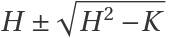:

 In:=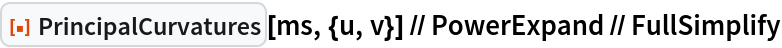Out=In:=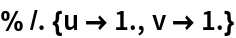Out=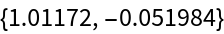The principal curvatures are equal to the eigenvalues of the Weingarten matrix:

 In:=Out=In:=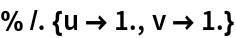Out=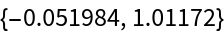If the principal curvatures have the same sign everywhere, that means there are only elliptic points on the surface:

 In:=Out=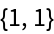Principal curvatures with different signs mean that there are only hyperbolic points on the surface:

 In:=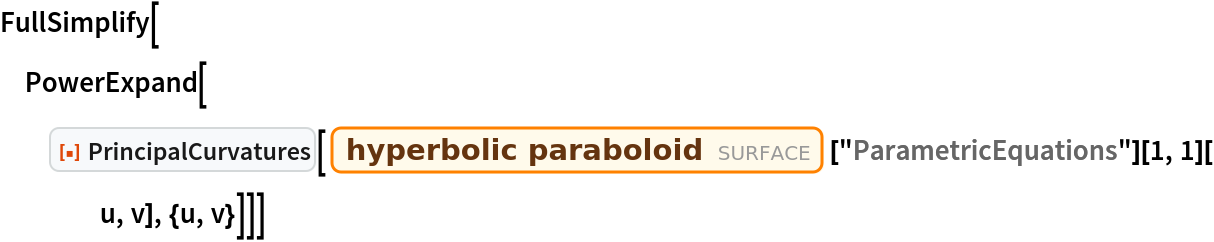Out=In:=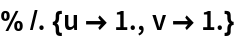Out=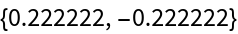When at least one principal curvature is equal to zero everywhere, there are only parabolic points on the surface:

 In:=Out=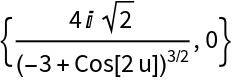When both are equal to zero, there are only planar points on the surface:

 In:=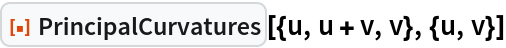Out=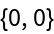Enrique Zeleny

## Version History

• 1.1.0 – 05 August 2021
• 1.0.0 – 17 April 2020